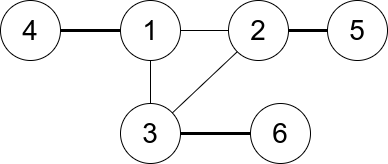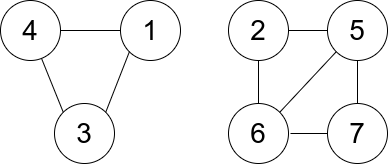# GeetCode Hub

You are given an undirected graph. You are given an integer `n` which is the number of nodes in the graph and an array `edges`, where each `edges[i] = [ui, vi]` indicates that there is an undirected edge between `ui` and `vi`.

A connected trio is a set of three nodes where there is an edge between every pair of them.

The degree of a connected trio is the number of edges where one endpoint is in the trio, and the other is not.

Return the minimum degree of a connected trio in the graph, or `-1` if the graph has no connected trios.

Example 1:```Input: n = 6, edges = [[1,2],[1,3],[3,2],[4,1],[5,2],[3,6]]
Output: 3
Explanation: There is exactly one trio, which is [1,2,3]. The edges that form its degree are bolded in the figure above.
```

Example 2:```Input: n = 7, edges = [[1,3],[4,1],[4,3],[2,5],[5,6],[6,7],[7,5],[2,6]]
Output: 0
Explanation: There are exactly three trios:
1) [1,4,3] with degree 0.
2) [2,5,6] with degree 2.
3) [5,6,7] with degree 2.
```

Constraints:

• `2 <= n <= 400`
• `edges[i].length == 2`
• `1 <= edges.length <= n * (n-1) / 2`
• `1 <= ui, vi <= n`
• `ui != vi`
• There are no repeated edges.

class Solution { public int minTrioDegree(int n, int[][] edges) { } }